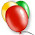## Thursday, January 23, 2003

### The Lambda Calculus, Part 1

One cannot celebrate Alonzo Church, part of our Celebration of Geniuses without talking about his creation, the Lambda Calculus, a way to describe functions and functional evaluation with a very simple description and incredible power.

As an example, consider the square function, square(x)=x*x. Suppose we don't care about the name and just want to talk about the function in the abstract. The lambda calculus gives us the syntax for such discussions. We express the square function as

λx.x*x
This is a function that takes one argument and returns its square. For example
λx.x*x(5) = 25
Also note that the use of x is not important. The following is also the square function.
λy.y*y
So now let us formally define the syntax of the Lambda Calculus. The alphabet consists of an infinite list of variables v0, v1, the abstractor "λ", the separator "." and parentheses "(" and ")". The set of lambda terms is the smallest set such that
1. Every variable is a lambda term.
2. If M is a lambda term then (λx.M) is a lambda term.
3. If M and N are lambda terms then MN is a lambda term.
We will sometimes leave off parentheses when the meaning is clear. Examples of lambda terms are xx, λx.xx, λx.λy.yx.
Free variables are those not closed off by a λ. For example in λy.xy the variable x is free and y is bound.

We use the notation M[x:=E] means replace every occurrence in the lambda term M of the free variable x by the lambda term E. There should not be any free variables in E that are bound in M as this could cause confusion.

There are two basic operations:
(renaming variables formally called α-conversion) λx.M to λy.M[x:=y] where y does not occur in M
(function evaluation formally called β-reduction) λx.M(E) to M[x:=E]

Church and Rossner have shown that if you have a complicated lambda-term it does not matter what order the β-reduction operations are applied.

What can you do with just these simple operations? You get the same power as Turing machines! It's instructive to see this connection and we'll go over the proof over several posts in the future.

#### 1 comment:

1.